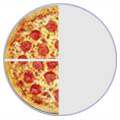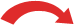# Simplifying FractionsTo simplify a fraction, divide the top and bottom by the highest number that
can divide into both numbers exactly.

## Simplifying Fractions

Simplifying (or reducing) fractions means to make the fraction as simple as possible.

Why say four-eighths (48 ) when we really mean half ( 12) ?

 48 = 24 = 12 (Four-Eighths) (Two-Quarters) (One-Half)==## How do I Simplify a Fraction ?

There are two ways to simplify a fraction:

## Method 1

Try to exactly divide (only whole number answers) both the top and bottom of the fraction by 2, 3, 5, 7 ,... etc, until we can't go any further.

### Example: Simplify the fraction 24 108 :

 ÷ 2 ÷ 2 ÷ 324108   =   1254   =   627   =   29÷ 2 ÷ 2 ÷ 3

That is as far as we can go. The fraction simplifies to 2 9

### Example: Simplify the fraction 10 35 :

Dividing by 2 doesn't work because 35 can't be exactly divided by 2 (35/2 = 17½)

Likewise we can't divide exactly by 3 (10/3 = 3 1 3 and also 35/3=11 2 3 )

No need to check 4 (we checked 2 already, and 4 is just 2×2).

But 5 does work!

 ÷ 51035   =   27÷ 5

That is as far as we can go. The fraction simplifies to 2 7

Notice that after checking 2 we didn't need to check 4 (4 is 2×2)?

We also don't need to check 6 when we have checked 2 and 3 (6 is 2x3).

In fact, when checking from smallest to largest we use prime numbers:

2, 3, 5, 7, 11, 13, 17, 19, 23, 29, 31, 37, ...

## Method 2

Divide both the top and bottom of the fraction by the Greatest Common Factor (you have to work it out first!).

### Example: Simplify the fraction 812 :

The largest number that goes exactly into both 8 and 12 is 4, so the Greatest Common Factor is 4.

Divide both top and bottom by 4:

 ÷ 4812   =   23÷ 4

That is as far as we can go. The fraction simplifies to 2 3

## Simplifying Fractions Automatically

OK, there is a third method, use this tool:## Fractions on the Number Line

Also ... see an animation of Fractions on the Number Line where you can see many common fractions and their simpler version.

We also have a chart of fractions with the simplest fraction highlighted.